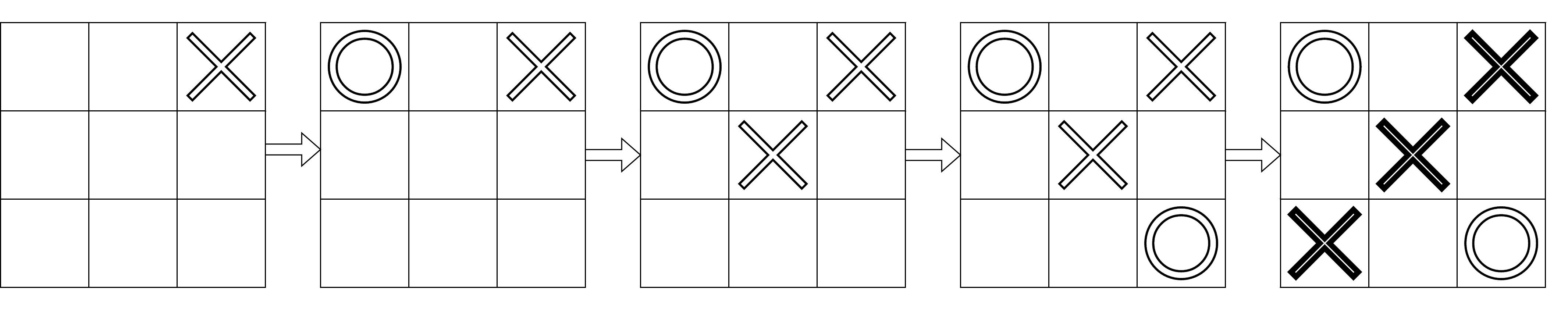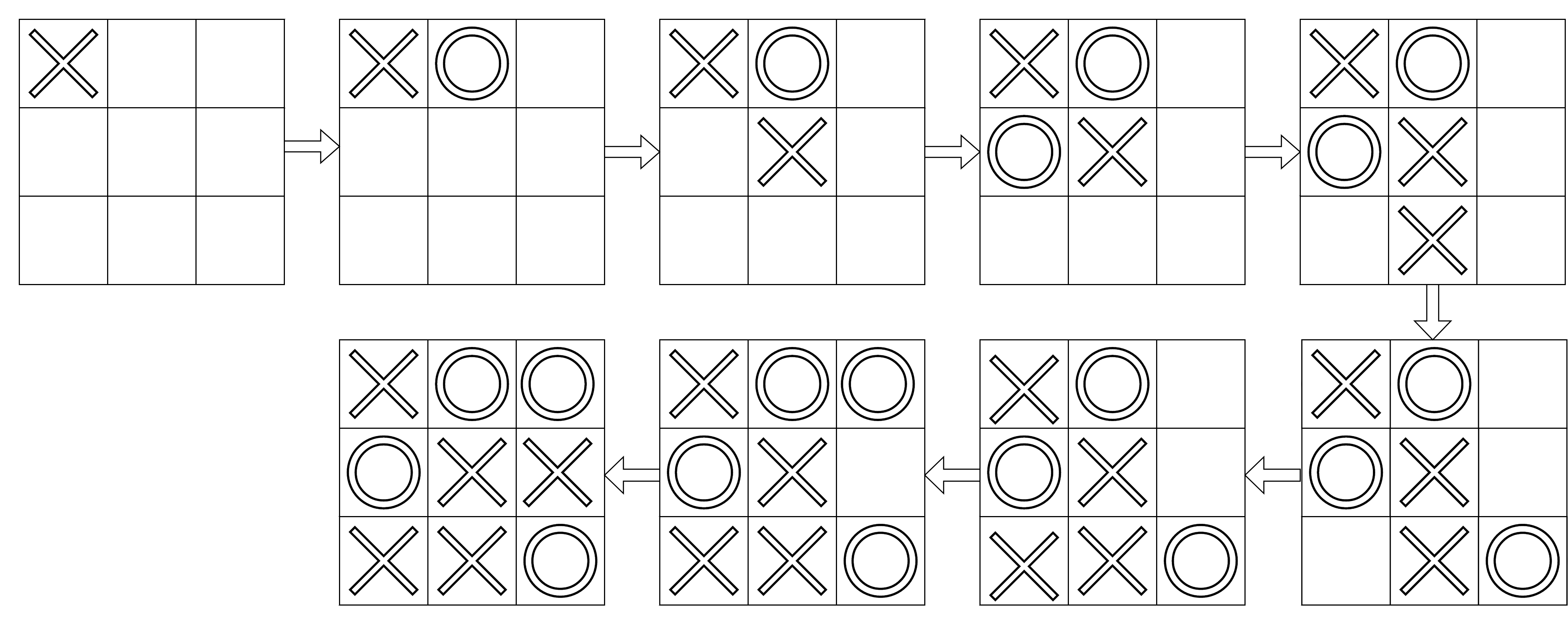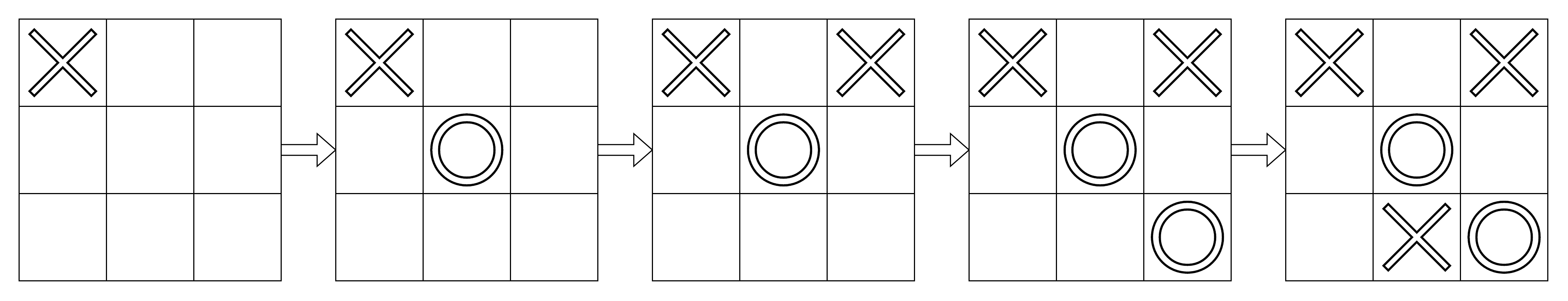Problem title
Difficulty
Avg time to solve

Minimum Swaps to Group All 1's Together
Easy
15 mins
Ninja And Bombs
Easy
15 mins
Palindrome Permutation
Easy
15 mins
Longest Path In Directed Graph
Moderate
30 mins
Multilevel Inheritance
Easy
--
Recursive Multiply
Moderate
20 mins
Maximum Difference Of Zeros And Ones In A Binary String
Moderate
15 mins
Find Duplicates Using Bit Array
Moderate
10 mins
Sum Paths
Moderate
30 mins
Tic-Tac-Toe Winner
Easy
20 mins5

# Tic-Tac-Toe Winner

Difficulty: EASY
Avg. time to solve
20 min
Success Rate
80%

Problem Statement

#### Example :

``````n = 5, moves = {{0,2}, {0,0}, {1,1}, {2,2}, {2,0}}
``````#### Note :

``````1. The array ‘moves’ doesn’t contain any repeating positions, and all positions are valid.
2. The array ‘moves’ follows all the rules of tic-tac-toe.
3. You do not need to print anything; it has already been taken care of. Just implement the function
``````

#### Input format :

``````The first line of input contains an integer ‘T’ denoting the number of test cases.

The first line of each test case contains an integer ‘N’ denoting the size of the array ‘moves’.

The next ‘N’ lines of each test case contain two space-separated integers denoting a tuple of the array ‘moves’.
``````

#### Output format :

``````For each test case, if there is a winner, print their name, i.e., ‘player1’ or ‘player2’. Otherwise, depending on the grid’s final state, print ‘uncertain’ or ‘draw’ (without quotes).
``````

#### Constraints :

``````1 <= T <= 1000
1 <= N <= 9
| moves[i] | = 2
0 <= moves[i][j] <= 2

Where ‘moves[i][j]’ represents elements in the array ‘moves’.

Time limit: 1 second
``````

#### Sample input 1 :

``````2
9
0 0
0 1
1 1
1 0
2 1
2 2
2 0
0 2
1 2
5
0 0
1 1
0 2
2 2
2 1
``````

#### Sample output 1 :

``````draw
uncertain
``````
##### Explanation of sample input 1 :
``````Test Case 1:
````````````There is no row, column, or diagonal with three same characters after performing the given nine moves. Hence there is no winner. With no more moves to make (all grid positions are marked), the game ends in a draw. So, the answer is ‘draw’.

Test Case 2:
````````````There is no row, column, or diagonal with three same characters after performing the given five moves. Hence there is no winner as of now. With four grid positions remaining unmarked, the winner of the game is uncertain. So, the answer is ‘uncertain’.
``````

#### Sample input 2 :

``````2
6
0 0
1 1
2 2
0 2
1 0
2 0
5
0 1
1 2
2 1
1 0
1 1
``````

#### Sample output 2 :

``````player2
player1
``````Console